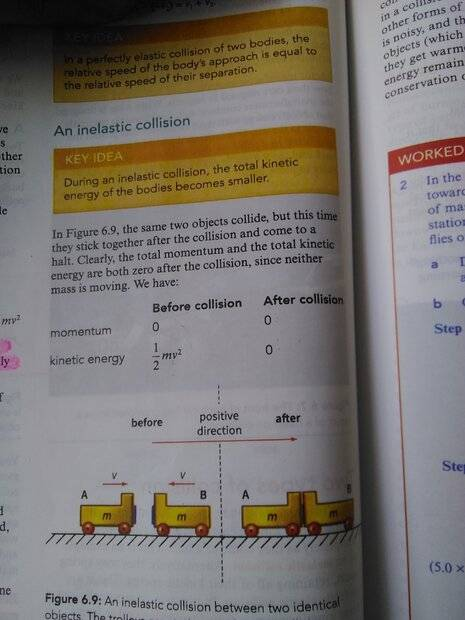# Inelastic collisions with constant momentum

haha0p1
Homework Statement:
The total momentum before the collision in an inelastic collisions is 0, but the total kinetic energy before the collision is 1/2mv². Calculate how the total kinetic energy before collision is 1/2mv².
Relevant Equations:
Ek=1/2mv²
Kinetic energy before collision =1/2 mv² + 1/2 mv² = mv² (since energy is a scalar quantity, the direction does not matter). Kindly tell why am I not getting the required answer i.e: 1/2 mv². Am I doing the calculation wrong?Last edited by a moderator:

Homework Helper
Hi,

I agree with your calculation. An unfortunate error in the book.

##\ ##

•haha0p1
Homework Helper
Gold Member
2022 Award
It's interesting that the book specifies the "same object" or "identical" objects, where all that is required kinematically is objects of the same mass!

haha0p1
It's interesting that the book specifies the "same object" or "identical" objects, where all that is required kinematically is objects of the same mass!
Truee

Homework Helper
Gold Member
It's interesting that the book specifies the "same object" or "identical" objects, where all that is required kinematically is objects of the same mass!
I think it's economy of words. "Identical objects" is shorter than "objects of the same mass" and conveys the idea of symmetry. "Identical" becomes relatively conciser when the masses also carry equal charges.

•PeroK